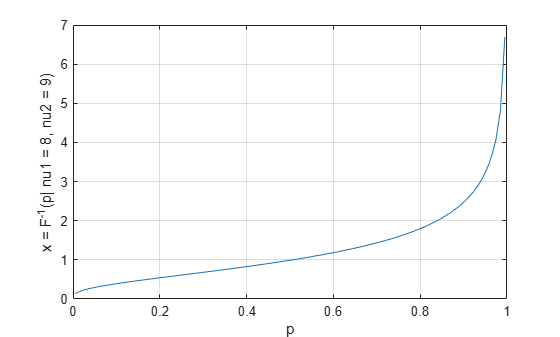# finv

F inverse cumulative distribution function

## Syntax

``x = finv(p,nu1,nu2)``

## Description

example

````x = finv(p,nu1,nu2)` returns the inverse cumulative distribution function (icdf) of the F distribution with degrees of freedom `nu1` (numerator) and `nu2` (denominator), evaluated at the probability values in `p`.```

## Examples

collapse all

Compute the inverse cdf values evaluated at the probability values in `p` for an F distribution with degrees of freedom `nu1` and `nu2`.

```p = linspace(0.005,0.995,100); nu1 = 8; nu2 = 9; x = finv(p,nu1,nu2);```

Plot the inverse cdf.

```plot(p,x) grid on xlabel("p") ylabel("x = F^{-1}(p| nu1 = " + num2str(nu1)... + ", nu2 = " + num2str(nu2) + ")")```Consider two independent random samples of size n1 and n2 drawn from normal distributions. The variance ratio of the samples has an F distribution with n1–1 and n2–1 degrees of freedom. Use the inverse cdf of the F distribution to calculate a range [0 `r95`] so that the variance ratio has a 95% probability of being in this range.

```rng default % For reproducibility n1 = 100; n2 = 105; p = 0.95; r = finv([0 p],n1-1,n2-1)```
```r = 1×2 0 1.3874 ```

Generate two random samples from the standard normal distribution and calculate their variance ratio.

```s1 = randn([n1 1]); s2 = randn([n2 1]); r12 = var(s1)/var(s2)```
```r12 = 1.3749 ```

The variance ratio `r12` is in the range [0 `r95`].

Consider two independent random samples of size `n1` and `n2` drawn from normal populations with unknown variances `var1` and `var2`. The samples have variances `v1` and `v2`. Use the inverse cdf of the F distribution to calculate a 95% confidence interval for the ratio `var1`/`var2`.

Enter the sample sizes and variances, and calculate the ratio of sample variances.

```n1 = 122; n2 = 124; v1 = 1.3; v2 = 1.2; r = v1/v2```
```r = 1.0833 ```

The ratio of sample variances is `r`.

Compute the 95% confidence interval for the population variance ratio `var1`/`var2`.

```pCI = 95; p = (1+pCI/100)/2; rLow = v1/v2/finv(p,n1-1,n2-1)```
```rLow = 0.7586 ```
`rHigh = v1/v2*finv(p,n2-1,n1-1)`
```rHigh = 1.5479 ```

The probability that the population variance ratio `var1`/`var2 `is in the range [rLow rHigh] is 0.95.

## Input Arguments

collapse all

Probability values at which to evaluate the inverse of the cdf (icdf), specified as a scalar value or an array of scalar values, where each element is in the range `[0,1]`.

• To evaluate the icdf at multiple values, specify `p` using an array.

• To evaluate the icdfs of multiple distributions, specify `nu1` and `nu2` using arrays.

If one or more of the input arguments `p`, `nu1`, and `nu2` are arrays, then the array sizes must be the same. In this case, `finv` expands each scalar input into a constant array of the same size as the array inputs.

Example: `[0.1,0.5,0.9]`

Data Types: `single` | `double`

Number of degrees of freedom in the numerator of the F distribution function, specified as a positive scalar value or an array of positive scalar values.

• To evaluate the icdf at multiple values, specify `p` using an array.

• To evaluate the icdfs of multiple distributions, specify `nu1` and `nu2` using arrays.

If one or more of the input arguments `p`, `nu1`, and `nu2` are arrays, then the array sizes must be the same. In this case, `finv` expands each scalar input into a constant array of the same size as the array inputs.

Example: [ 8 7 9]

Data Types: `single` | `double`

Number of degrees of freedom in the denominator of the F distribution function, specified as a positive scalar value or an array of positive scalar values.

• To evaluate the icdf at multiple values, specify `p` using an array.

• To evaluate the icdfs of multiple distributions, specify `nu1` and `nu2` using arrays.

If one or more of the input arguments `p`, `nu1`, and `nu2` are arrays, then the array sizes must be the same. In this case, `finv` expands each scalar input into a constant array of the same size as the array inputs.

Example: [ 7 6 10]

Data Types: `single` | `double`

## Output Arguments

collapse all

inverse cdf values evaluated at the probabilities in `p`, returned as a scalar value or an array of scalar values. `x` is the same size as `p`, `nu1`, and `nu2` after any necessary scalar expansion. Each element in `x` is the inverse cdf value of the distribution specified by the corresponding elements in `nu1` and `nu2`, evaluated at the corresponding probability in `p`

collapse all

### F Distribution

The F inverse function is defined in terms of the F cumulative distribution function (cdf) as

`$x={F}^{-1}\left(p|{\nu }_{1},{\nu }_{2}\right)=\left\{x:F\left(x|{\nu }_{1},{\nu }_{2}\right)=p\right\}$`

where

`$p=F\left(x|{\nu }_{1},{\nu }_{2}\right)=\underset{0}{\overset{x}{\int }}\frac{\Gamma \left[\frac{\left({\nu }_{1}+{\nu }_{2}\right)}{2}\right]}{\Gamma \left(\frac{{\nu }_{1}}{2}\right)\Gamma \left(\frac{{\nu }_{2}}{2}\right)}{\left(\frac{{\nu }_{1}}{{\nu }_{2}}\right)}^{\frac{{\nu }_{1}}{2}}\frac{{t}^{\frac{{\nu }_{1}-2}{2}}}{{\left[1+\left(\frac{{\nu }_{1}}{{\nu }_{2}}\right)t\right]}^{\frac{{\nu }_{1}+{\nu }_{2}}{2}}}dt$`

The ν values are the degrees of freedom, and Γ( · ) is the Gamma function. The result x is the solution of the integral equation where you supply the probability p.

• `finv` is a function specific to the F distribution. Statistics and Machine Learning Toolbox™ also offers the generic function `icdf`, which supports various probability distributions. To use `icdf`, specify the probability distribution name and its parameters. Note that the distribution-specific function `finv` is faster than the generic function `icdf`.